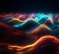## IFFT of Non causal signal?Started by 1 month ago3 replieslatest reply 1 month ago164 views

Hi,

I have a frequency domain data (that is non-causal). How can I calculate the IFFT in MATLAB so that I can visualize the impulse response behavior of t<0.

Regards,

Rahul

[ - ]I can only guess as what you are after:

ifft is meant for frequency domain data, not time domain data.

May be you mean ifftshift(x), this will take you to t<0

for example, a fir filter from given amplitude:

%%%%%%%%%%%%%%%%%%%%%%%%%%%%%%%%%%%%%%%%%%%%%%%

amp = [1, ones(1,150), zeros(1,1000),  ones(1,150)]; %frequency domain

h = real(ifft(amp)); %time domain

h = ifftshift(h);

[ - ]Dear Kaz,

Apologies for the typo, yes, you are right. I have a complex-valued frequency domain data (from a transmission line operating at 0 to 20 GHz) and I am interested in calculating its impulse response in time-domain so to evaluate its time-delay.

BR,

Rahul

[ - ]